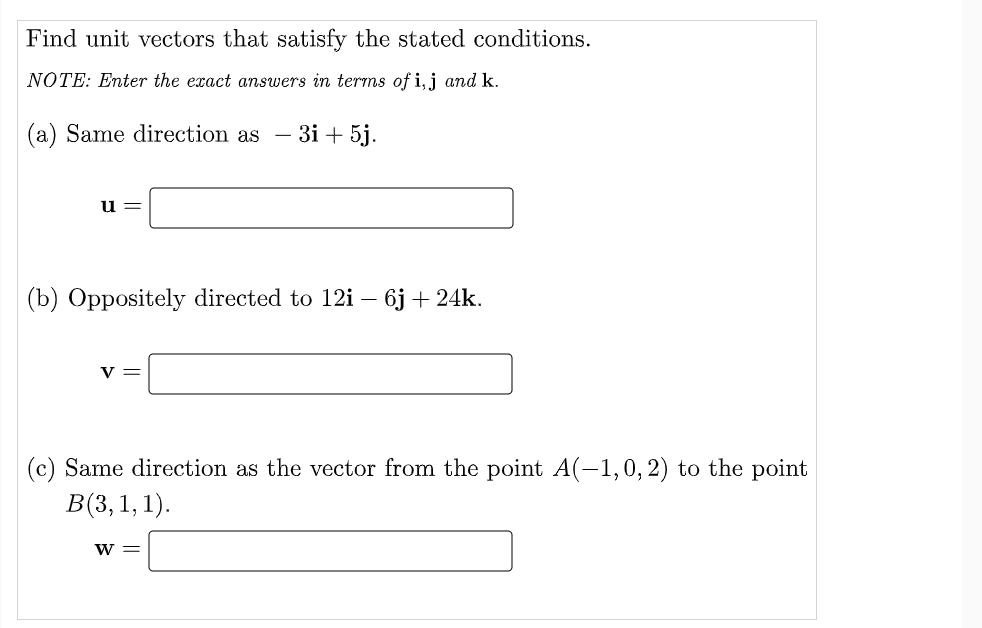# Question Find unit vectors that satisfy the stated conditions. NOTE: Enter the exact answers in terms of i, j and k. (a) Same direction as – 3i + 5j. u = (b) Oppositely directed to 12i – 6j + 24k. — V = (c) Same direction as the vector from the point A(-1,0, 2) to the point B(3,1,1). W =